Home | | Maths 12th Std | Exercise 7.10: Choose the correct or the most suitable answer

# Exercise 7.10: Choose the correct or the most suitable answer

Choose the correct or the most suitable answer from the given four alternatives : Mathematics - Applications of Differential Calculus

Choose the correct or the most suitable answer from the given four alternatives :

1. The volume of a sphere is increasing in volume at the rate of 3ŽĆ cm3 / sec .

The rate of change of its radius when radius is 1/2 cm

(1) 3 cm/s

(2) 2 cm/s

(3) 1 cm/s

(4) 1/2 cm/s2. A balloon rises straight up at 10 m/s. An observer is 40 m away from the spot where the balloon left the ground. The rate of change of the balloonŌĆÖs angle of elevation in radian per second when the balloon is 30 metres above the ground.3. The position of a particle moving along a horizontal line of any time t is given by s (t) = 3t2 ŌłÆ 2t ŌłÆ 8 . The time at which the particle is at rest is

(1) t = 0

(2) t = 1/3

(3) t = 1

(4) t = 34. A stone is thrown up vertically. The height it reaches at time t seconds is given by x = 80t ŌłÆ16t2 . The stone reaches the maximum height in time t seconds is given by

(1) 2

(2) 2.5

(3) 3

(4) 3.5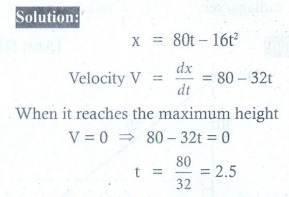5. The point on the curve 6= x3 + 2 at which y-coordinate changes 8 times as fast as x-coordinate is

(1) (4,11)

(2) (4, ŌłÆ11)

(3) (ŌłÆ4,11)

(4) (ŌłÆ4, ŌłÆ11)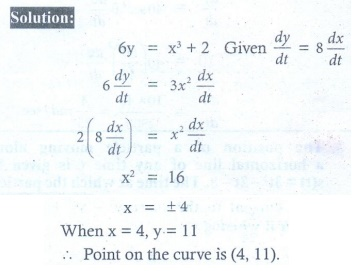6. The abscissa of the point on the curve f (x) = ŌłÜ(8ŌłÆ2x) at which the slope of the tangent is ŌłÆ0.25 ?

(1) ŌłÆ8

(2) ŌłÆ4

(3) ŌłÆ2

(4) 07. The slope of the line normal to the curve f (x) = 2 cos 4x at x = ŽĆ/12 is

(1) ŌłÆ4ŌłÜ3

(2) ŌłÆ4

(3) ŌłÜ3 /  12

(4) 4ŌłÜ38. The tangent to the curve y2 ŌłÆ xy + 9 = 0 is vertical when

(1) y = 0

(2) y = ┬▒ ŌłÜ3

(3) y = 1/2

(4) y = ┬▒39. Angle between y2 = x and x2 = y at the origin is

(1) tanŌłÆ1 (3/4)

(2) tan-1 (4/3)

(3) ŽĆ/2

(4) ŽĆ/4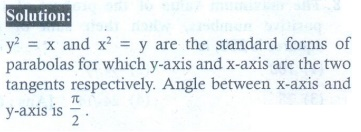10. The value of the limitor limx ŌåÆ0 ( cot x ŌĆō 1/x )is

(1) 0

(2) 1

(3) 2

(4) Ōł×11. The function sin4 x + cos4 x is increasing in the interval12. The number given by the RolleŌĆÖs theorem for the function x3 ŌłÆ 3x2 , x Ōłł[0, 3] is

(1) 1

(2) ŌłÜ2

(3) 3/2

(4) 213. The number given by the Mean value theorem for the function 1/x , x Ōłł[1, 9] is

(1) 2

(2) 2.5

(3) 3

(4) 3.514. The minimum value of the function | 3 ŌłÆ x | + 9 is

(1) 0

(2) 3

(3) 6

(4) 9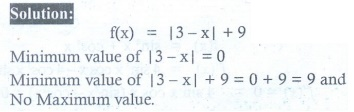15. The maximum slope of the tangent to the curve y = ex sin x , x Ōłł[0, 2ŽĆ ] is at

(1) x = ŽĆ/4

(2) x = ŽĆ/2

(3) x = ŽĆ

(4) x = 3ŽĆ/2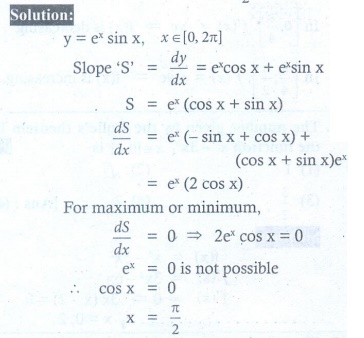16. The maximum value of the function x2 eŌłÆ2x , x > 0 is

(1) 1/e

(2) 1/2e

(3) 1/e2

(4) 4/e417. One of the closest points on the curve x2 ŌłÆ y2 = 4 to the point (6, 0) is

(1) (2, 0)

(2) (ŌłÜ5,1)

(3) (3, ŌłÜ5 )

(4) (ŌłÜ13, ŌłÆŌłÜ3)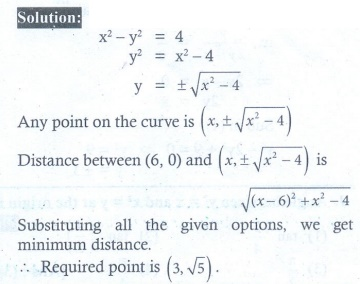18. The maximum value of the product of two positive numbers, when their sum of the squares is 200, is

(1) 100

(2) 25ŌłÜ7

(3) 28

(4) 24ŌłÜ1419. The curve y = ax4 + bx2 with ab > 0

(1) has no horizontal tangent

(2) is concave up

(3) is concave down

(4) has no points of inflection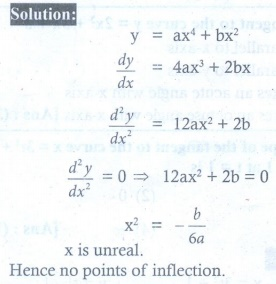20. The point of inflection of the curve y = (x ŌłÆ1)3 is

(1) (0, 0)

(2) (0,1)

(3) (1, 0)

(4) (1,1)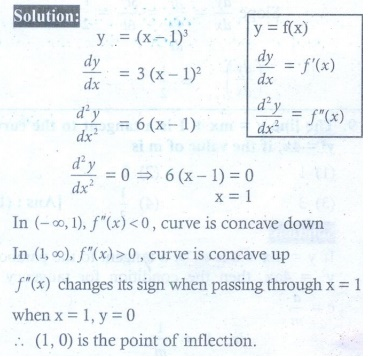Tags : Applications of Differential Calculus | Mathematics , 12th Maths : UNIT 7 : Applications of Differential Calculus
Study Material, Lecturing Notes, Assignment, Reference, Wiki description explanation, brief detail
12th Maths : UNIT 7 : Applications of Differential Calculus : Exercise 7.10: Choose the correct or the most suitable answer | Applications of Differential Calculus | Mathematics